# Private Variables in ARMA 3 SQF

Private variables and scopes are pretty simple in ARMA 3 scripting. The problem is—as it often is—that the documentation isn't very clear. Confounding matters further, SQF uses dynamic scoping—rather than the more common and easier to understand conventions of static scoping.

## Blocks

A block is any piece of code surrounded by braces, or compiled using the compile function. You can think of braces as a shortcut for compiling a string of code. For example, these two snippets of code are practically identical:

```if (_naughty) then { player setDamage 1 };
```
```if (_naughty) then (compile "player setDamage 1");
```

The result of both the braced code block and the compile call is to create a CODE object—the only difference between them is when exactly the code is compiled. A CODE object—like other objects such as players, vehicles and strings—may be assigned to variables, passed to functions, and placed into arrays.

The code inside a block isn't executed when the block is created. To make the code run, the CODE object created by the block needs to be called, either by using the call or spawn operators, or by using it in control statements such as if or forEach:

```_someCode = { player setDamage 1; };

// The player is always still alive here.

if (1 < 2) then _someCode;

// Now, not so much.
```

## Scopes

When a CODE object begins to run—because you called it or used it in a control statement—the SQF engine creates a scope. When it finishes running—either by getting to the end or by exiting early with exitWith or one of the break commands—the engine automatically destroys the scope.

A scope is created every time a block is called, even if it is the same block being called multiple times. This is what makes private variables—which are stored in scopes—private.

For example, if you spawn a CODE object that uses a private variable, twice:

```_someCode = { private "_a"; _a = random 10; };

[] spawn _someCode;
[] spawn _someCode;
```

The engine creates two independent scopes, which each contain different instances of a variable called _a.

Scopes can be stacked on top of each other. When a scope is created, the engine usually stacks it on top of the current scope, which is the scope that called the CODE object:

```_naughty = true;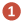_someCode = {
private "_d";
_d = random 1;
player setDamage _d;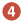};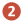if (_naughty) then
{
// The if resulted in a scope being created for this
// code block; when _someCode is called the engine will
// create another scope and stack it on top of this one: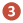call _someCode;
};
```

As the above example is executed, the scopes look like this: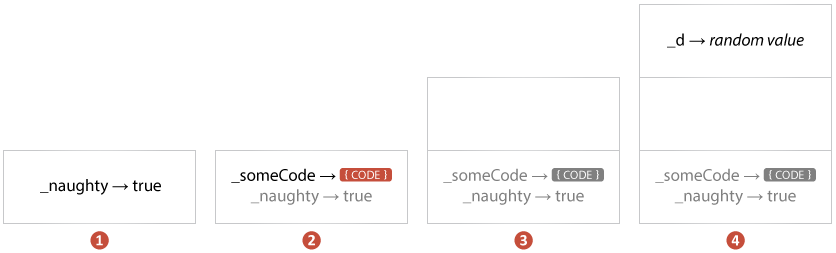Stacking of scopes is how the engine lets private variables wander out into other scopes. Consider this code:

```private "_value"; _value = random 1;

if (_value < 0.5) then { _value = 0; }
```

If scopes didn't stack, the { _value = 0; } code block would not have access to update the private variable created in the outer scope.

When a scope is destroyed—because the code finished running—it is also taken off the stack of scopes.

## The Rules

Scopes are created and stacked based on these rules:

• The call operator, and the control statements if, switch, while, for forEach, waitUntil and try create a scope for the code blocks they invoke, and stack it on top of the current scope.
• The spawn and execVM operators—as well as event handlers that call code blocks—create a new isolated scope that is not stacked on any other scope. This is why spawned code starts with no private variables—other than the _this variable that is injected in—and has no access to the private variables of the code that spawned it.

When you read a private variable—as in hint _someVariable:

• The stack of scopes is searched from the current (topmost) scope downwards until the private variable is found. If it is not found, the expression result is nil.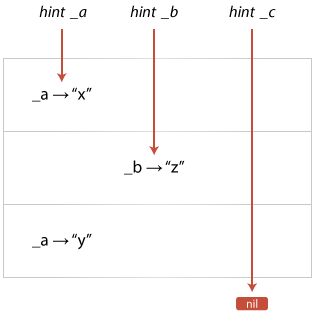When you update a private variable—as in _someVariable = "hello":

• The stack of scopes is searched from the current (topmost) scope downwards until the private variable is found. If it is found, it is updated in the same scope it was found in. If it is not found, one is created in the current (topmost) scope.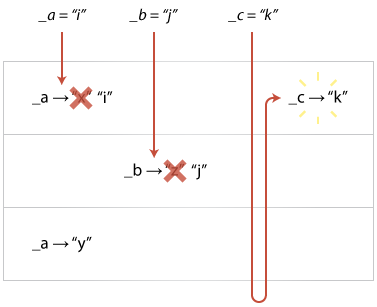Two things can go wrong with this rule. The first is that the current scope is often not the scope you want your private variable in:

```if (_someCondition) then
{
_myLocal = 1;
}
else
{
_myLocal = 2;
};

hint str _myLocal; // error; "_myLocal" is not defined.
```

The second thing that can go wrong is that you don't always know if a private variable is already defined in a lower scope. If it is, the assignment will update it when it probably shouldn't have:

```badFunction =
{
// oops, forgot to use private:
_x = getPos player select 0;
_y = getPos player select 1;

_x + _y
};

{

hint str _x; // prints the "x" coordinate of the player; eek!
}
forEach [1, 2, 3, 4];
```

To deal with both issues, the following rules exist:

• When private and params create a private variable, they always do so within the current scope, ignoring any other scopes and variables in the stack of scopes.
• When forEach creates _x and _forEachIndex, and when for "_name" creates a variable called _name, they do so within the called scopes, not the calling scope.
• When call creates _this, it does so in the called scope, not the calling scope.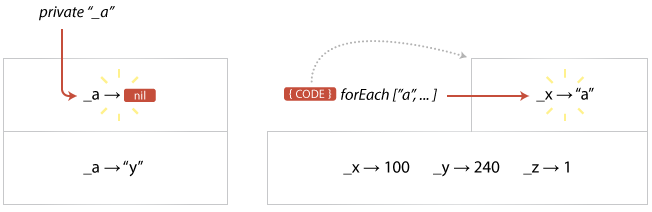Commands like params and forEach work by assigning values to private variables in a specific scope. The private command also does this; but it assigns the special nil value to the variable. You can see this happening with this example:

```private "_a";

_a = 1;

hint str isNil "_a"; // hints "false"

if (true) then
{
private "_a";

hint str isNil "_a"; // hints "true"
}
```

What happens when a private variable exists in more than one scope?

The rules stated above for reading and updating private variables explain this case; the topmost scope that contains the private will be read from or updated, and the lower scopes containing the same private are ignored; until, that is, the upper scope is destroyed. At that point the lower private variable becomes visible again:

```private "_a";

_a = 1;

if (true) then
{
private "_a";

_a = 2;
}

hint str _a; // hints "1"
```

## Assigning nil

Assigning nil to a private variable doesn't actually undefine the variable. nil is a value, just like 1 is a value, and the rules for assigning it—to private variable at least—are exactly the same. You can see this with the following example:

```private "_a";

_a = 1;

if (true) then
{
private "_a";

_a = 2;
_a = nil;

_a = 3;
};

hint str _a; // hints "1"
```

If _a = nil removed the private variable from the scope, you would expect the subsequent _a = 3 to be applied to the outer scope. It isn't—though—and _a continues to be a private variable within the inner scope.

However, global variables are handled differently. If you assign nil to a global variable, it is considered a special case and the variable name is removed from the symbol table of the namespace (which can be verified by making a call to allVariables on the namespace).

## Always, Without Exception, Use Private

One of the consequences of the rules for private variables is that any function you call can read—and change—every private variable from the bottommost scope of the first .sqf file executed (with execVM or spawn) all the way up through the call stack to itself. Take this script for example:

```test = { hint str [_a, _b]; };

private "_a";
_a = 1;
call test; // hints [1, any]

private "_b";
_b = 2;
call test; // hints [1, 2]
```

This is true even for compiled functions, like bis_fnc_diagLoop; if the function reads or writes a private variable without using private first, there is a risk it will overwrite the variable in some unrelated scope.

The only time you might be able to avoid using private is when:

• The code is in the outermost scope of a script that is exclusively invoked by spawn or execVM; and,
• You will never, ever, forget that this code can't safely be invoked from an arbitrary call; and,
• You know that not you, nor any other person, will at any time in the future copy and paste this code into another routine or file that is invoked with call.

In other words, never.

## Lexical Scoping and Dynamic Scoping

One of the key things to remember about SQF scoping is that a CODE object has no idea where it was originally created. At first glance, the following looks like the inner function would have the private variable _a defined:

```if (true)
{
private "_a";

_a = 1;

inner =
{
hint str _a;
};
};

call inner;
```

In SQF—however—it doesn't. The scope that _a is created in is destroyed once the if block finishes, and the CODE object assigned to the global inner has no idea that it was created in that scope. Instead, the only private variables available to a called CODE object come from the scopes of the code that called it. This is called dynamic scoping.

This isn't the case in other languages. In many other languages, the equivalent to CODE objects also include references to local variables that are physically (lexically) close to where they were created—rather than where they are used. This is called lexical scoping, and is often preferred because it makes more sense to humans.

## A Silver Lining

A common trick (or "idiom") in ARMA 3 scripting is special private variables, where the engine calls your code with some variables already defined. For example, forEach does it with _x, and onMapSingleClick does it with _pos. There are quite a few in ARMA 3, and for more you can refer to Killzone Kid's comprehensive list of all of them.

The same thing is possible from user code, due entirely because of the rules of SQF's dynamic typing. For example, if you were writing a framework that allowed the user to hook framework generated events, you could call the user function with your own special variables:

```fwk_fnc_addEventHandler =
{
fwk_eventHandlers pushBack _this;
};

fwk_fnc_invokeNukeDetonationEvent =
{
[] spawn
{

_event = "nukeDetonation";
```{ hint str [_event, _radius]; } call fwk_fnc_addEventHandler;